# KSEEB Solutions for Class 10 Maths Chapter 9 Polynomials Additional Questions

Students can Download Class 10 Maths Chapter 9 Polynomials Additional Questions, Notes Pdf, KSEEB Solutions for Class 10 Maths helps you to revise the complete Karnataka State Board Syllabus and score more marks in your examinations.

## Karnataka State Syllabus Class 10 Maths Chapter 9 Polynomials Additional Questions

I. Multiple Choice Questions:

Question 1.
The degree of polynomial is x + 2
a. 2
b. 1
c. 3
d. 4
b. 1Question 2.
The degree of a quadratic polynomial is
a. 0
b. 1
c. 2
d. 3
c. 2

Question 3.
The degree polynomial of a bi-quadratic
a. 0
b. 2
c. 4
d. 1
c. 4

Question 4.
The standard polynomial. form of a linear
a. ax + c
b. ax2 + c
c. ax2 + bx + c = 0
d. ax3 + bx2 + cx + d = 0
a. ax + c

Question 5.
The standard form of a quadratic equation.
a. ax2 + bx + c = 0
b. ax + c = 0
c. ax2 + c = 0
d. ax3 + bx + c = 0
a. ax2 + bx + c = 0

Question 6.
A polynomial of degree 3 is called
a. a linear polynomial
c. a cubic polynomial
c. a cubic polynomialQuestion 7.
The value of px. = x2 – 3x – 4, x = -1
a. 1
b. – 4
c. 0
d. – 3
c. 0

Question 8.
The degree of the polynomial x4 + x3 is
a. 2
b. 3
c. 5
d. 4
d. 4

Question 9.
The number of zeroes of linear polynomial at most is
a. 0
b. 1
c. 2
d. 3
b. 1

Question 10.
The degree of polynomial x + 2. x + 1. is
a. 1
b. 3
c. 4
d. 2
d. 2

Question 11.
The degree of a zero polynomial is
a. not defined
b. 1
c. 2
d. 3
a. not defined

Question 12.
The zeroes of the polynomial x3 -4x are
a. 0, ± 2
b. 0, ± 1
c. 0, ± 3
d. 0, 0
a. 0, ± 2

Question 13.
The zeroes of the polynomials, t2 – 15 are.
a. ± $$\sqrt{15}$$
b. ± $$\sqrt{5}$$
c. ± $$\sqrt{3}$$
d. ± 3
a. ± $$\sqrt{15}$$Question 14.
Dividend is equal to.
a. divisor × quotient + remainder
b. divisor × quotient
c. divisor × quotient – remainder
d. divisor × quotient × remainder
a. divisor × quotient + remainder

Question 15.
If the divisor is x2 and quotient is x while the number remainder is 1, then the dividend is.
a. x2
b. x
c. x3
d. x3 + 1
d. x3 + 1

Question 16.
What is the co-efficient of the first term of the quotient when 2x2 + 2x + 1 is divided by x + 2?
a. 1
b. 2
c. 3
d. – 2
b. 2

Question 17.
The zeroes of the polynomial x2 – 3x – 4 are
a. 4, – 1
b. 4, 1
c. – 4, 1
d.- 4, – 1
a. 4, – 1

Question 18.
If f(x) = x2 – 1 the value of f(2) is
a. 1
b. 3
c. 4
d. 0
b. 3

Question 19.
If f(x) = 8 f(x) is called
a. Constant polynomials
b. linear polynomials
d. Cubic polynomials
a. Constant polynomialsQuestion 20.
A cubic polynomial has at most
a. 1 zeroes
b. 2 zeroes
c. 3 zeroes
d. 4 zeroes.
c. 3 zeroes

Question 1.
Find the value of P(x) = x2 + 2x – 5 at x = 1
P(x) = x2 + 2x – 5
P (1) = (1)2 + 2(1) – 5 = 1 + 2 – 5
= 3 – 5
P(1) = – 2

Question 2.
If x = 1 is a zero of the Polynomial f(x) = x3 – 2x2 + 4x + K, write the value of K.
f(x) = x3 – 2x2 + 4x + K x = 1
f(1) = (1)3 – 2(1)2 + 4(1) + K
f(1) = 0 zero of the polynomial
0 = 1 – 2 + 4 + K
0 = 3 + K
K = – 3

Question 3.
If α and β are zeroes of Polynomials P(x) = x2 – 5x + 6, then find the value of α + β – 3αβ.
α + β = $$\frac{-b}{a}=\frac{-(-5)}{1}$$ = 5 and
αβ = $$\frac{c}{a}=\frac{6}{1}$$ = 6
α + β – 3αβ
= 5 – 3(6)
= 5 – 18 = -13
α + β – 3αβ = – 13Question 4.
Find the zeroes of the Polynomial P(x) = 4x2 – 12x + 9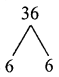P(x) = 4x2 – 12x + 9
4x2 – 6x – 6x + 9 = 0
2x(2x – 3) – 3(2x – 3) = 0
(2x – 3) (2x – 3) = 0
x = $$\frac{3}{2}$$, $$\frac{3}{2}$$

Question 5.
If 1 is a zero of the Polynomial P(x) = ax2 – 3(a – 1) x – 1, then find the value of a.
P(x) = ax2 – 3(a – 1) x – 1
∴ x = 1
P(1) = a(1)2 – 3(a – 1) 1 – 1
P(1) = a – 3a + 3 – 1
0 = – 2a + 2
2a = 2
a = $$\frac{2}{2}$$ = 1
a = 1

Question 6.
Write the degree of the Polynomial 3x3 – x4 + 5x + 3
3x3 – x4 + 5x + 3
– x4 + 3x3 + 5x + 3
∴ degree of the Polynomial is 4

Question 7.
Write the standard form of a cubic Polynomial.
ax3 + bx3 + cx + d.

Question 8.
Find the zero of the Polynomial , P(x) = a2x, a ≠ 0
P(x) = a2x
a2x = 0
x = $$\frac{0}{a^{2}}$$
x = 0

Question 9.
If α and β are the zeroes of the polynomial 4x2 + 3x + 7, then find the value of $$\frac{1}{\alpha}+\frac{1}{\beta}$$Question 1.
Find the zeroes of the following quadratic polynomials x2 + 4x + 4.
f(x) = x2 + 4x + 4
0 = x2 + 2x + 2x + 4
0 = x(x + 2) + 2(x + 2)
(x + 2) (or) (x + 2) = 0
x + 2 = 0 (or) x + 2 = 0
x = – 2 x = – 2.
Zeroes of the Polynomials x2 + 4x + 4 are – 2 and – 2Question 2.
If x = 1 is a zero of the polynomial f(x) = x3 – 2x2 + 4x + K, find the value of K.
f(x) = x3 – 2x2 + 4x + K
∴ x = 1
f(x) = (1)3 – 2(1)2 + 4(1) + K
0 = 1 – 2(1) + 4 + K [f(1) = 0]
0 = 1 – 2 + 4 + K.
K + 3 = 0
K = – 3

Question 3.
For what value of K, – 4 is a zero of the polynomial x2 – x – (2K + 2)?
f(x) = x2 – x – (2K + 2) and f(x) = 0
x = – 4
f(- 4) = (- 4)2 (- 4) – (2K + 2)
0 = 16 + 4 – 2K – 2
20 – 2 – 2K = 0 ⇒ 18 = 2K
K = $$\frac{18}{2}$$ ⇒ K = 9

Question 4.
Divide p(x) by g(x) in each of the following cases and verify division algorithm.
P(x) = x2 + 4x + 4, g(x) = x + 2
x2 + 4x + 4 ÷ x + 2q(x) = x + 2 and r(x) = 0

Question 5.
On dividing the polynomial. P(x) = x3 – 3x2 + x + 2 by a polynomial g(x) the quotient and remainder were (x – 2) and (- 2x + 4) respectively. Find g(x).
P(x) = x3 – 3x2 + x + 2
q(x) = (x – 2); r(x) = (- 2x + 4)
g(x) = ?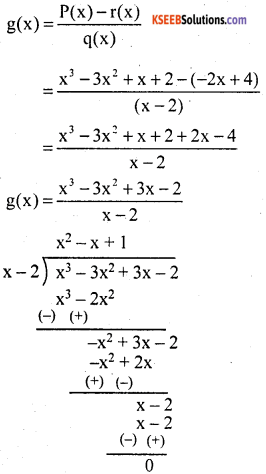g(x) = x2 – x + 1; r(x) = 0
∴ The divisor is x2 – x + 1Question 6.
What must be subtracted from x3 + 5x2 + 5x + 8 so that the resulting polynomial is exactly divisible by x2 + 3x – 2?
P(x) = x3 + 5x2 + 5x + 8
g(x) = x2 + 3x – 2
P(x) = g(x) q(x) + r(x)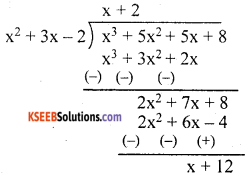q(x) = x + 2; r(x) = x + 12
If we subtract x + 12 from x3 + 5x2 + 5x + 8 it will be exactly divisible by x2 + 3x – 2

Question 7.
What should be added to (x4 – 1) so that it’ is exactly divisible by (x2 + 2x + 1)?
P(x) = x4 – 1 g(x) = x2 + 2x + 1
x4 + 0x3 + 0x3 + 0x – 1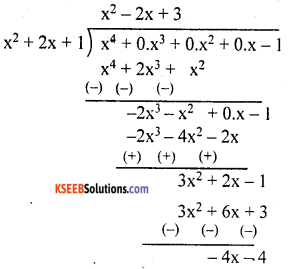r(x) = – 4x – 4
– {r(x)} = {4x + 4}

Question 8.
If the polynomials 2x3 + ax2 + 3x – 5 and x3 + x2 – 4x – a leave the same remainder when divided by x – 1 find the value of a.
x – 1 = 0 [∴ g(x) = (0)]
x = 1
P(x) = 2x3 + ax2 + 3x – 5
P(1) = 2(1)3 + a(1)3 + 3(1) – 5
P(1) = 2 + a + 3 – 5
P(1) = a ➝ (1)
g(x) = x3 + x2 – 4x – a
g(1) = (1)3 + (1)2 – 4(1) – a
= 1 + 1 – 4 – a
g(x) = – 2 – a ➝ (2)
g(1) = g(1) [∴ Polynomials are leave same remainder]
a = – 2 – a
a + a = – 2
2a = – 2
a = $$\frac{-2}{2}$$
a = – 1

Question 9.
If (x3 + ax2 – bx + 10) is divisible by x3 – 3x + 2, find the values of a and b.
P(x) = x3 + ax2 – bx + 10
g(x) = x2 – 3x + 2
x2 – 2x – x + 2 = 0
x(x – 2) – 1(x – 2) = 0
(x – 2) (x – 1) = 0
x = 2 and x = 1
P(x) = x3 + ax2 – bx + 10
P(x) = (1)3 + a(1)2 – b(1) + 10
= 1 + a – b + 10
0 = a – b + 11
a – b = – 11 ➝ (1)
P(2) = (2)3 + a(2)2 – b(2) + 10
P(2) = 8 + 4a – 2b – 10
0 = 4a – 2b + 18
4a – 2b = – 18 ➝ (2)
Multiply equation (1) by 4 and subtract with equation (2)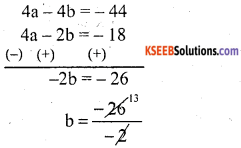b = 13
Put b = 13 in equation (1)
a – b = – 11
a – 13 = – 11
a = – 11 + 13
a = 2Question 10.
If both x – 2 and x – $$\frac{1}{2}$$ are factors of ax2 + 5x + b show that a = b.
P(x) = ax2 + 5x2 + b
x – 2 = 0
x = 2
P(x) = ax2 + 5x + b
P(2) = a(2)2 + 5(2) + b
0 = 4a + 10 + b ➝ (1)
P(x) = ax2 + 5x + b
x – $$\frac{1}{2}$$ = 0
x = $$\frac{1}{2}$$
P(x) = ax2 + 5x + b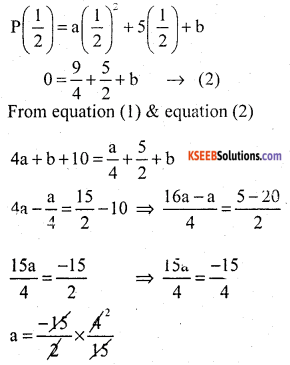a = – 2 ➝ (3)
Put a = – 2 in equation (1)
4a – 10 + b = 0
4(- 2) + 10 + b = 0
– 8 + 10 + b = 0
2 + b = 0
b = -2 ➝ (4)
From (3) and (4)
a = b.

Question 11.
If α and β are the zeroes of the quadratic polynomials f(x) = 2x2 – 5x + 7, find a polynomial whose zeroes are 2α + 3β and 3α + 2β.
Since α and β are the zeroes of the quadratic polynomial f(x) = 2x2 – 5x + 7.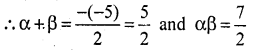Let S and P denote respectively the sum and product of the zeroes of the required polynomial.
Then, S = (2α + 3β) + (3α + 2β)
= 5(α + β) = 5 × $$\frac{5}{2}=\frac{25}{2}$$ and
P = (2α + 3β) (3α + 2β)
⇒ P = (6α2 + 6β2 + 13αβ)
= 6α2 + 12αβ + αβ + 6β2
⇒ P = 6(α2 + β2 + 2αβ + αβ
= 6(α + β)2 + αβ
= 6(α2 + β2 + 2αβ) + αβ
= 6(α + β)2 + αβ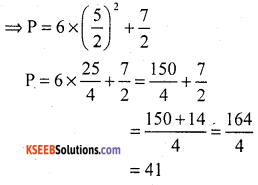Hence the required polynomial g(x) is given by
g(x) = K(x2 – 5x + P)
or g(x) = K$$\left(x^{2}-\frac{25}{2} x+41\right)$$, where K and any non zero real number.

Question 12.
If α and β are the zeroes of the polynomial. 6y2 – 7y + 2, find a quadratic polynomial whose zeroes are $$\frac{1}{\alpha}$$ and $$\frac{1}{\beta}$$.
Let P(y) = 6y2 – 7y + 2Question 13.
If one zero of the polynomial 3x2 – 8x + 2k + 1 and seven times the other, find the value of k.
Let α and β be the zeroes of the polynomial. Then as per question β = 7α.
Now sum of zeroes = α + β = α + 7αQuestion 14.
If one zero of polynomial (a2 + 9)x2 + 13x + 6a and reciprocal of the other, find the value of a.
Let one zero of the given polynomial be α
Then, the other zero and $$\frac{1}{\alpha}$$
∴ Product of zeroes = α × $$\frac{1}{\alpha}$$ = 1
But, as per the given polynomial product of Zeroes = $$\frac{6 a}{a^{2}+9}$$
∴ $$\frac{6 a}{a^{2}+9}$$ = 1 ⇒ a2 + 9 = 6a
⇒a2 – 6a + 9 = 0 ⇒ (a – 3)2 = 0
⇒ a – 3 = 0 ⇒ a = 3
Hence a = 3.

Question 15.
Find the zeroes of the polynomial f(x) = x3 – 5x2 – 2x + 24 if it is given that the product of its two zeroes is 12.
Let α, β and γ be the zeroes of polynomial f(x) such that αβ = 12Putting αβ = 12 in αβγ = – 24 we get
12γ = – 24 ⇒ γ = $$\frac{-24}{12}$$ = – 2
Now α + β + γ = 5 ⇒ α + β – 2 = 5
⇒ α + β = 7
⇒ α = 7 – β
∴ αβ =12
⇒ (7 – β) β = 12
⇒ 7β = β2 = 12
⇒ β2 – 7β + 12 = 0
⇒ β2 – 3β – 4β – 12 = 0
⇒β(β -3) – 4(β – 3) = 0
⇒(β -4)(β – 3) = 0
⇒ β = 4 or β = 3
α = 3 or α = 4.

Question 16.
If α, β, γ be zeroes of polynomial 6x3 + 3x2 – 5x +1, then find the value of α– 1 + β– 1 + γ– 1